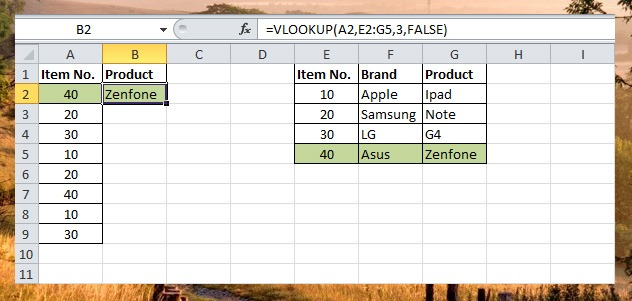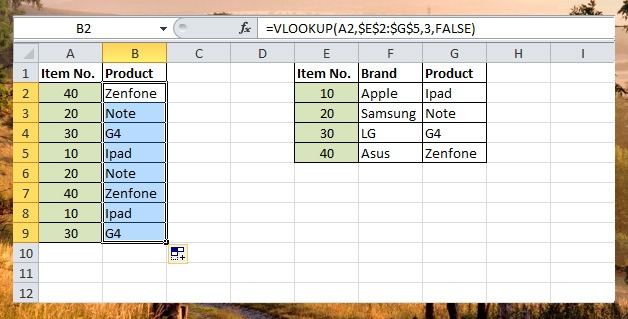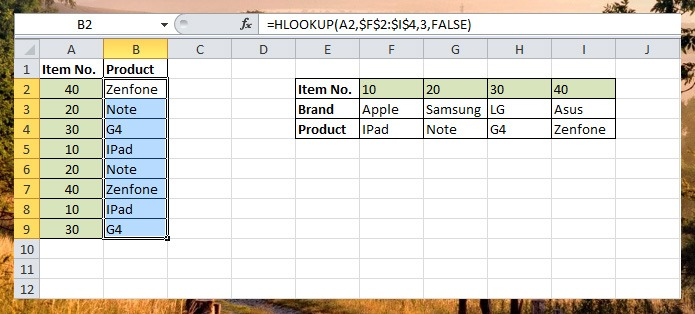# Lookup Functions

Lookup functions in Excel are divided into two, VLookup (Vertical Lookup) function which look for the value in the leftmost column of the range of cells you specify in table-array and the HLookup (Horizontal Lookup) function look for value at the topmost row of the table array specified.

VLookup Function

VLookup function is used to find value from the leftmost column of the table-array and returns the value in the same row from different column you specify.

Take at look at the example below, VLookup function will search for the same value entered in A2, from the leftmost column in Table E2:G5, when vlookup finds exact match it will return the value from the same row from the third column which is set in the third argument.To make the Table E2:G5 absolute reference, we will add \$ symbol before the Column letter and row number, so it will become  \$E\$2:\$G\$5. Now that the reference table is fixed we can now drag the formula down from B2 to B9.HLookup Function

HLookup function work similar to vlookup but instead of searching for value from leftmost column it will search at the top row of the table-array.

We will revise the reference table we use in vlookup function to see the similarities.1.bambie araneta
•Efren Nolasco